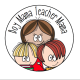# Teacher Mama: 5 Fun Ways to Practice Math FactsHow easily can you recall the answers to basic math facts?  If someone were to say to you, “What is 6×9?” How long would take you to figure out the answer?

Now imagine being a third grader and your class is being introduced to multiple digit addition or subtraction which requires a great deal of basic math fact recall. You do not know your basic facts. How long will it be before everyone else in the class has mastered the skill and you are still trying to figure out basic math facts?  That would not be good.  In fact, that was me in elementary school. I had not mastered my basic facts and each year fell further behind. It was not until I was a teacher myself, that I learned the importance of math fact fluency for higher-order math skills.

Here are a few facts about math fluency:

• “Youngsters who have not mastered whole number arithmetic by the end of 4th grade are at risk of later becoming remedial students in mathematics.”  (Tom Loveless, Director of the Brown Center on Education Policy at the Brookings Institution)
• If a student is struggling to remember basic facts, less of that child’s thinking capacity can be devoted to higher level concepts than a student who can effortlessly recall the answers to basic facts.
• The NCTM’s latest guidelines state that “second graders should be able to quickly recall basic addition and subtraction facts and fourth graders must have quick recall of multiplication and division facts.”

Why is learning math facts important:

• Experts agree that the ability to recall basic math facts fluently is necessary for students to attain higher-order math skills such as multiple-digit addition and subtraction, long division and fractions.
• Lack of math fact fluency has been found to impede participation in math class discussions, successful mathematics problem-solving and even the development of everyday life skills.
• Rapid math-fact retrieval has been shown to be a strong predictor of performance on mathematics achievement tests.

The information shared above comes from the following article.  If you want to read more about the importance of math fact fluency,  please click the following link:  Math Fluency: Scholastic.com

So now we know how important math fact fluency is for children.  Here are 5 fun ways to practice math facts.  All activities listed use the following number cards.

Pick a Fact Mats

Choose a practice mat to work on. Place the cards face down.  Chose two cards and place them on the ma and solve the problem. For addition, place one card on each “addend” space. For subtraction, place the larger number on the “minuend” space and the smaller number on the “subtrahend” place.  The answer to the math problem can be shown with the correct card as shown below OR can be done verbally.

Note: The words addend, minuend and subtrahend are not words that young children are required to know. But as I have said before, kids love big words so have some fun with them. The words sum and difference will be words they encounter more and more so playing around with them can only help!Say and Find Mat

Give your child a blank Say and Find Mat (see below). Choose a fact set to practice (ie +3) and write it in the “math fact box” at the top left.  Write all the sums (see the +3 column below) on the mat or have your child do it.  Then read a fact (ie 2+3) and have your child locate the answer on the mat.  He can then put an X through (circle it, highlight it) the correct answer. Continue until all the numbers have been crossed off.  This game can be played with subtraction, multiplication or division.  You can also try it with sight words!Math Fact Card Mat

This is a two player game.  Place the cards face down.  Choose addition or subtraction and place the sign in the middle of the board (see below).  Player one draws a card and puts it on the board. Player 2 then draws a card and places it on the board. Players take turns reading the math sentence and solving the problem.The player that solves this problem above would say, “Four plus eight equals twelve.”  Be careful if you are practicing subtraction!  The player who draws the bigger number has to put it first unless you want to practice negative numbers which would be a great challenge for strong math students!

Math Fact Bean Bag Toss

This game will get your child up and moving when practicing math facts.  Cut out the larger numbers circles and spread them out on the floor in front of you.  Choose to practice addition or subtraction.  Use a bean bag, rock, or other small object and throw them onto two numbers.   Then read the problem and solve it!  See the example below.

Note:  I made three bean bags- two plain colored bean bags and one with an + sign on one side and an – sign on the other.  With each toss, you can also toss the +/- bean bag to see what kind of problem the player will solve.  You can also use a coin in the same way if you label both sides or use the big +/- circles above.Player tosses the bean bags and they land on 7 and 3.  Player then says, “Seven plus three equals ten.”Player tosses the bean bags and they land on 5 and 1.  Player then says, “Five minus one equals four.”

* * * * * * * * * * * *

Math Practice Fun from Boy Mama Teacher Mama

© Boy Mama Teacher Mama 2012## 8 thoughts on “Teacher Mama: 5 Fun Ways to Practice Math Facts”

1.1978rebecca

Brilliant ideas. I love the bean bag toss.

I have pinned this to The Sunday Showcase board

•boymamateachermama

Thank you!!

2.Paula Kluth

love it!

•boymamateachermama

Thanks Paula!